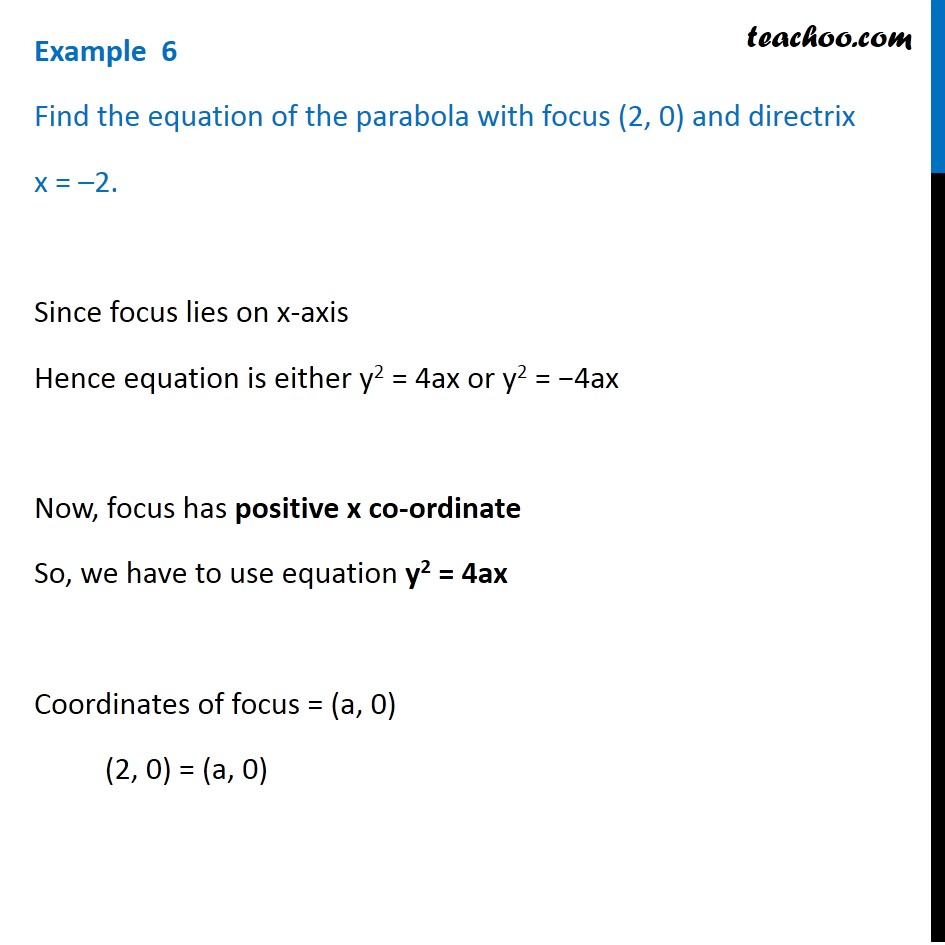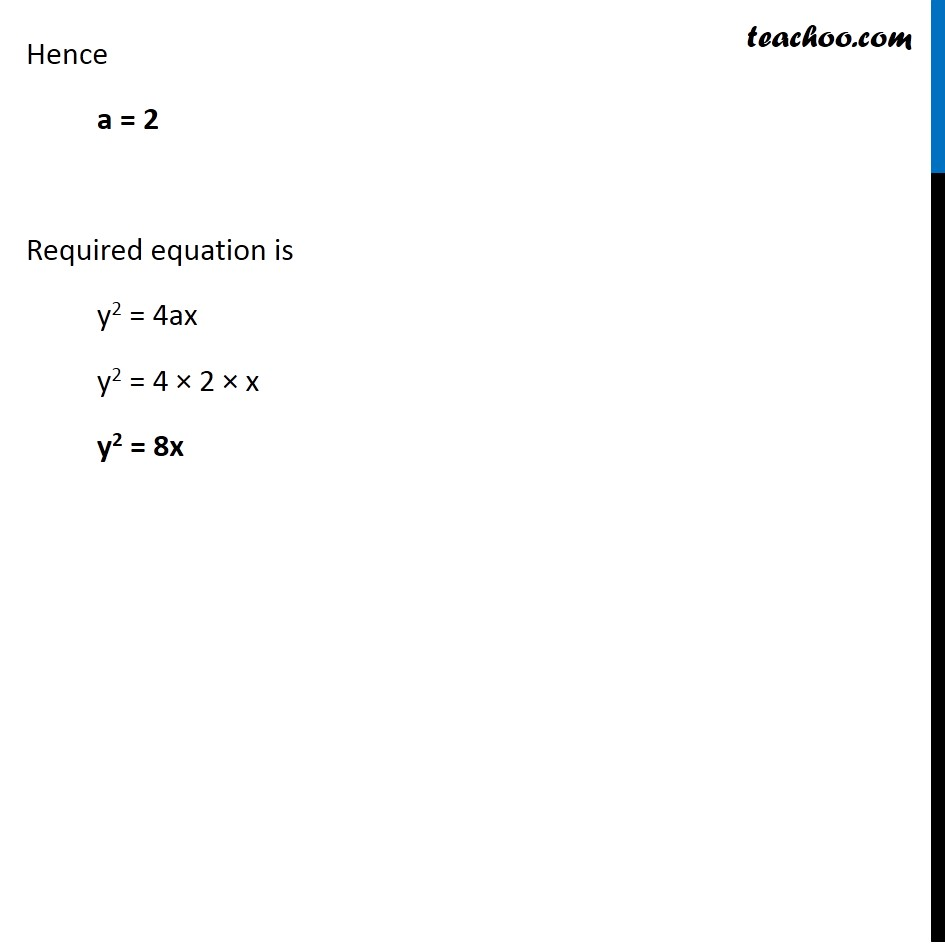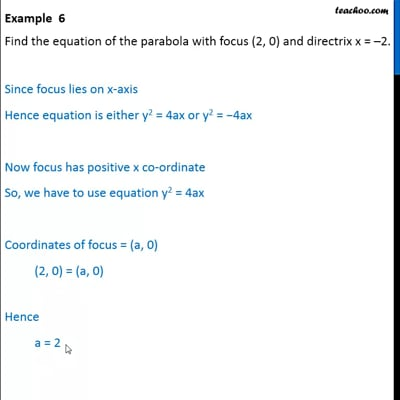Examples

Chapter 11 Class 11 Conic Sections
Serial order wiseThis video is only available for Teachoo black users

Solve all your doubts with Teachoo Black (new monthly pack available now!)

### Transcript

Example 6 Find the equation of the parabola with focus (2, 0) and directrix x = –2. Since focus lies on x-axis Hence equation is either y2 = 4ax or y2 = −4ax Now, focus has positive x co-ordinate So, we have to use equation y2 = 4ax Coordinates of focus = (a, 0) (2, 0) = (a, 0) Hence a = 2 Required equation is y2 = 4ax y2 = 4 × 2 × x y2 = 8x